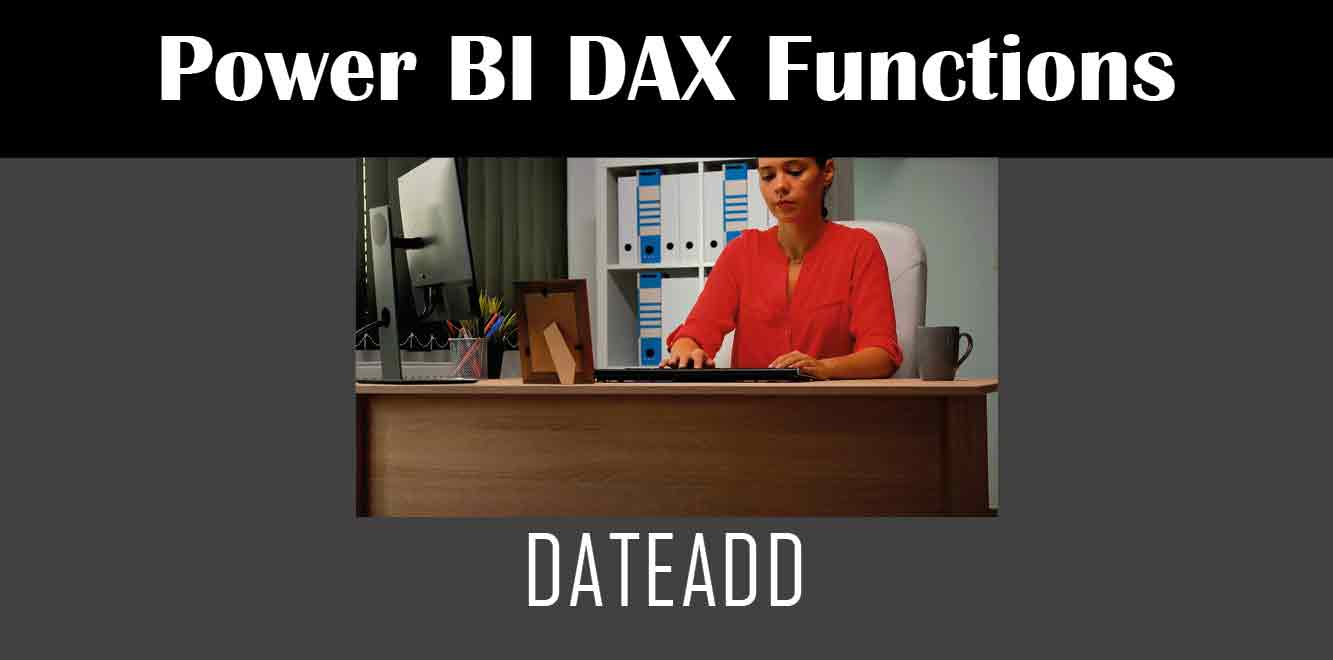## Y## What is the Power Fx DateAdd Function?

The Power Fx DateAdd function is a powerful function that allows you to add or subtract a specified number of units from a date value. This function is commonly used to manipulate dates in Power Apps.

## Syntax of the Power Fx DateAdd Function

The syntax of the Power Fx DateAdd function is as follows:

``` DateAdd(startDate, numberOfUnits, unit) ```

– `startDate`: This is the starting date value that you want to manipulate.

– `numberOfUnits`: This is the number of units that you want to add or subtract from the starting date value. This value can be positive or negative.

– `unit`: This is the unit of time that you want to add or subtract. This can be any of the following values: “day”, “week”, “month”, “quarter”, or “year”.

## Examples of the Power Fx DateAdd Function

Let’s take a look at some examples of how to use the Power Fx DateAdd function in Power Apps.

### Example 1: Adding Days to a Date Value

Suppose you have a date value of “10/1/2021”, and you want to add 7 days to this value. You can use the following formula:

``` DateAdd(DateValue("10/1/2021"), 7, "day") ```

This formula will return the value “10/8/2021”, which is 7 days after the starting date.

### Example 2: Subtracting Months from a Date Value

Suppose you have a date value of “1/1/2022”, and you want to subtract 3 months from this value. You can use the following formula:

``` DateAdd(DateValue("1/1/2022"), -3, "month") ```

This formula will return the value “10/1/2021”, which is 3 months before the starting date.

### Example 3: Adding Quarters to a Date Value

Suppose you have a date value of “1/1/2022”, and you want to add 2 quarters to this value. You can use the following formula:

``` DateAdd(DateValue("1/1/2022"), 2, "quarter") ```

This formula will return the value “7/1/2022”, which is 2 quarters after the starting date.

In conclusion, the Power Fx DateAdd function is a powerful tool that allows you to manipulate dates in Power Apps. With the syntax and examples provided in this article, you should now be able to use this function with confidence in your Power Apps projects.